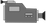Multiplying Binomials

Updated

Multiplying Binomials1. Multiplying a binomial with a binomial:2. Multiplying a binomial with a trinomial: